# Weierstrass E-function

(diff) ← Older revision | Latest revision (diff) | Newer revision → (diff)

in the classical calculus of variations

A function which isolates the main part of the increment of a functional as the extremal is varied, using a local (needle-shaped) variation for a given value of its derivative, at a given point of the extremal. In the case of the functionalthe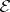-function has the form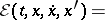(1)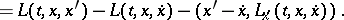If one introduces the function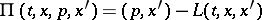(cf. Legendre transform; Pontryagin maximum principle), the-function assumes the formwhere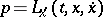. The general construction of functions analogous to the-function (1) consists of the following. Letbe a differentiable or convex function, defined on a Banach space, and let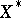be the dual space. If the function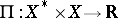is defined by the equation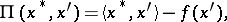where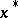is the derivativeofat(or the subdifferential element ifis convex), the function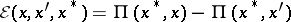is the-function constructed from. Ifis differentiable,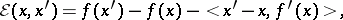(2)

i.e. the-function is the difference at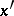betweenand the linear function tangent toat. A comparison of formulas (1) and (2) shows that in the classical calculus of variations the-function is obtained from the construction (2) with respect to the variables related to derivatives, while the variables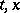play the role of parameters.

In the case of a functional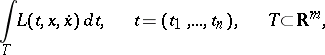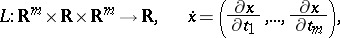in a multi-dimensional variational problem, the-function has the following form: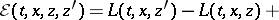In the case of the Lagrange problem with boundaries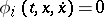and Lagrange multipliers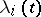,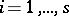, the-function has the form (1), in which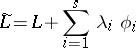has been substituted for.

The-function, first introduced in 1879 by K. Weierstrass , lies at the foundation of the theory of the calculus of variations (cf. Variational calculus). It is used in the formulation of necessary and (partially) sufficient conditions for an extremum (cf. Weierstrass conditions (for a variational extremum)), and serves to express the increment of a functional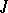on an extremal in the form of a finite integral (cf. Weierstrass formula).

An especially important role in variational calculus is played by smooth functionals in which, in a given parameter range,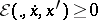for all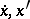, or, stronger, if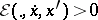for all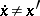. They are known as quasi-regular and regular or elliptic, respectively. For such functionals the Legendre condition and the necessary Weierstrass conditions (for a variational extremum) are invariably valid, as are the theorems of existence and regularity .

How to Cite This Entry:
Weierstrass E-function. Encyclopedia of Mathematics. URL: http://encyclopediaofmath.org/index.php?title=Weierstrass_E-function&oldid=19110
This article was adapted from an original article by V.M. Tikhomirov (originator), which appeared in Encyclopedia of Mathematics - ISBN 1402006098. See original article